This book is archived and will be removed July 6, 2022. Please use the updated version.

Discrete Random Variables

# Mean or Expected Value and Standard Deviation

The expected value is often referred to as the “long-term” average or mean. This means that over the long term of doing an experiment over and over, you would expect this average.

You toss a coin and record the result. What is the probability that the result is heads? If you flip a coin two times, does probability tell you that these flips will result in one heads and one tail? You might toss a fair coin ten times and record nine heads. As you learned in (Figure), probability does not describe the short-term results of an experiment. It gives information about what can be expected in the long term. To demonstrate this, Karl Pearson once tossed a fair coin 24,000 times! He recorded the results of each toss, obtaining heads 12,012 times. In his experiment, Pearson illustrated the Law of Large Numbers.

The Law of Large Numbers states that, as the number of trials in a probability experiment increases, the difference between the theoretical probability of an event and the relative frequency approaches zero (the theoretical probability and the relative frequency get closer and closer together). When evaluating the long-term results of statistical experiments, we often want to know the “average” outcome. This “long-term average” is known as the mean or expected value of the experiment and is denoted by the Greek letter μ. In other words, after conducting many trials of an experiment, you would expect this average value.

NOTE

To find the expected value or long term average, μ, simply multiply each value of the random variable by its probability and add the products.

A men’s soccer team plays soccer zero, one, or two days a week. The probability that they play zero days is 0.2, the probability that they play one day is 0.5, and the probability that they play two days is 0.3. Find the long-term average or expected value, μ, of the number of days per week the men’s soccer team plays soccer.

To do the problem, first let the random variable X = the number of days the men’s soccer team plays soccer per week. X takes on the values 0, 1, 2. Construct a PDF table adding a column x*P(x). In this column, you will multiply each x value by its probability.

Expected Value Table This table is called an expected value table. The table helps you calculate the expected value or long-term average.
x P(x) x*P(x)
0 0.2 (0)(0.2) = 0
1 0.5 (1)(0.5) = 0.5
2 0.3 (2)(0.3) = 0.6

Add the last column x*P(x) to find the long term average or expected value: (0)(0.2) + (1)(0.5) + (2)(0.3) = 0 + 0.5 + 0.6 = 1.1.

The expected value is 1.1. The men’s soccer team would, on the average, expect to play soccer 1.1 days per week. The number 1.1 is the long-term average or expected value if the men’s soccer team plays soccer week after week after week. We say μ = 1.1.

Find the expected value of the number of times a newborn baby’s crying wakes its mother after midnight. The expected value is the expected number of times per week a newborn baby’s crying wakes its mother after midnight. Calculate the standard deviation of the variable as well.

You expect a newborn to wake its mother after midnight 2.1 times per week, on the average.
x P(x) x*P(x) (xμ)2P(x)
0 P(x = 0) =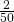(0)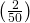= 0 (0 – 2.1)2 ⋅ 0.04 = 0.1764
1 P(x = 1) =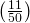(1)=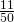(1 – 2.1)2 ⋅ 0.22 = 0.2662
2 P(x = 2) =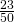(2)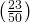=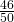(2 – 2.1)2 ⋅ 0.46 = 0.0046
3 P(x = 3) =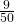(3)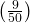=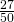(3 – 2.1)2 ⋅ 0.18 = 0.1458
4 P(x = 4) =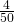(4)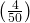=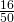(4 – 2.1)2 ⋅ 0.08 = 0.2888
5 P(x = 5) =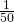(5)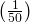=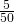(5 – 2.1)2 ⋅ 0.02 = 0.1682

Add the values in the third column of the table to find the expected value of X:
μ = Expected Value =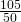= 2.1

Use μ to complete the table. The fourth column of this table will provide the values you need to calculate the standard deviation. For each value x, multiply the square of its deviation by its probability. (Each deviation has the format xμ).

Add the values in the fourth column of the table:

0.1764 + 0.2662 + 0.0046 + 0.1458 + 0.2888 + 0.1682 = 1.05

The standard deviation of X is the square root of this sum: σ =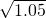≈ 1.0247

The mean, μ, of a discrete probability function is the expected value.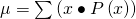The standard deviation, Σ, of the PDF is the square root of the variance.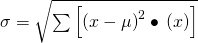When all outcomes in the probability distribution are equally likely, these formulas coincide with the mean and standard deviation of the set of possible outcomes.

Try It

A hospital researcher is interested in the number of times the average post-op patient will ring the nurse during a 12-hour shift. For a random sample of 50 patients, the following information was obtained. What is the expected value?

x P(x)
0 P(x = 0) =1 P(x = 1) =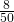2 P(x = 2) =3 P(x = 3) =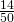4 P(x = 4) =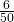5 P(x = 5) =Suppose you play a game of chance in which five numbers are chosen from 0, 1, 2, 3, 4, 5, 6, 7, 8, 9. A computer randomly selects five numbers from zero to nine with replacement. You pay ?2 to play and could profit ?100,000 if you match all five numbers in order (you get your ?2 back plus ?100,000). Over the long term, what is your expected profit of playing the game?

To do this problem, set up an expected value table for the amount of money you can profit.

Let X = the amount of money you profit. The values of x are not 0, 1, 2, 3, 4, 5, 6, 7, 8, 9. Since you are interested in your profit (or loss), the values of x are 100,000 dollars and −2 dollars.

To win, you must get all five numbers correct, in order. The probability of choosing one correct number is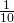because there are ten numbers. You may choose a number more than once. The probability of choosing all five numbers correctly and in order is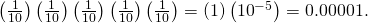Therefore, the probability of winning is 0.00001 and the probability of losing is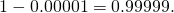The expected value table is as follows:

Αdd the last column. –1.99998 + 1 = –0.99998
x P(x) x*P(x)
Loss –2 0.99999 (–2)(0.99999) = –1.99998
Profit 100,000 0.00001 (100000)(0.00001) = 1

Since –0.99998 is about –1, you would, on average, expect to lose approximately ?1 for each game you play. However, each time you play, you either lose ?2 or profit ?100,000. The ?1 is the average or expected LOSS per game after playing this game over and over.

Try It

You are playing a game of chance in which four cards are drawn from a standard deck of 52 cards. You guess the suit of each card before it is drawn. The cards are replaced in the deck on each draw. You pay ?1 to play. If you guess the right suit every time, you get your money back and ?256. What is your expected profit of playing the game over the long term?

Suppose you play a game with a biased coin. You play each game by tossing the coin once. P(heads) =and P(tails) =. If you toss a head, you pay ?6. If you toss a tail, you win ?10. If you play this game many times, will you come out ahead?

a. Define a random variable X.

a. X = amount of profit

b. Complete the following expected value table.

x ____ ____
WIN 10____
LOSE ____ ____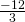b.

x P(x) xP(x)
WIN 10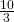LOSE –6c. What is the expected value, μ? Do you come out ahead?

c. Add the last column of the table. The expected value μ =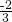. You lose, on average, about 67 cents each time you play the game so you do not come out ahead.

Try It

Suppose you play a game with a spinner. You play each game by spinning the spinner once. P(red) =, P(blue) =, and P(green) =. If you land on red, you pay ?10. If you land on blue, you don’t pay or win anything. If you land on green, you win ?10. Complete the following expected value table.

x P(x)
Red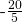BlueGreen 10

Like data, probability distributions have standard deviations. To calculate the standard deviation (σ) of a probability distribution, find each deviation from its expected value, square it, multiply it by its probability, add the products, and take the square root. To understand how to do the calculation, look at the table for the number of days per week a men’s soccer team plays soccer. To find the standard deviation, add the entries in the column labeled (xμ)2P(x) and take the square root.

x P(x) x*P(x) (xμ)2P(x)
0 0.2 (0)(0.2) = 0 (0 – 1.1)2(0.2) = 0.242
1 0.5 (1)(0.5) = 0.5 (1 – 1.1)2(0.5) = 0.005
2 0.3 (2)(0.3) = 0.6 (2 – 1.1)2(0.3) = 0.243

Add the last column in the table. 0.242 + 0.005 + 0.243 = 0.490. The standard deviation is the square root of 0.49, or σ == 0.7

Generally for probability distributions, we use a calculator or a computer to calculate μ and σ to reduce roundoff error. For some probability distributions, there are short-cut formulas for calculating μ and σ.

Toss a fair, six-sided die twice. Let X = the number of faces that show an even number. Construct a table like (Figure) and calculate the mean μ and standard deviation σ of X.

Tossing one fair six-sided die twice has the same sample space as tossing two fair six-sided dice. The sample space has 36 outcomes:

 (1, 1) (1, 2) (1, 3) (1, 4) (1, 5) (1, 6) (2, 1) (2, 2) (2, 3) (2, 4) (2, 5) (2, 6) (3, 1) (3, 2) (3, 3) (3, 4) (3, 5) (3, 6) (4, 1) (4, 2) (4, 3) (4, 4) (4, 5) (4, 6) (5, 1) (5, 2) (5, 3) (5, 4) (5, 5) (5, 6) (6, 1) (6, 2) (6, 3) (6, 4) (6, 5) (6, 6)

Use the sample space to complete the following table:

Calculating μ and σ.
x P(x) xP(x) (xμ)2P(x)
0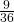0 (0 – 1)2=1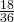(1 – 1)2= 0
2(1 – 1)2=Add the values in the third column to find the expected value: μ =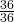= 1. Use this value to complete the fourth column.

Add the values in the fourth column and take the square root of the sum: σ =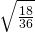≈ 0.7071.

On May 11, 2013 at 9:30 PM, the probability that moderate seismic activity (one moderate earthquake) would occur in the next 48 hours in Iran was about 21.42%. Suppose you make a bet that a moderate earthquake will occur in Iran during this period. If you win the bet, you win ?50. If you lose the bet, you pay ?20. Let X = the amount of profit from a bet.

P(win) = P(one moderate earthquake will occur) = 21.42%

P(loss) = P(one moderate earthquake will not occur) = 100% – 21.42%

If you bet many times, will you come out ahead? Explain your answer in a complete sentence using numbers. What is the standard deviation of X? Construct a table similar to (Figure) and (Figure) to help you answer these questions.

x P(x) x(Px) (xμ)2P(x)
win 50 0.2142 10.71 [50 – (–5.006)]2(0.2142) = 648.0964
loss –20 0.7858 –15.716 [–20 – (–5.006)]2(0.7858) = 176.6636

Mean = Expected Value = 10.71 + (–15.716) = –5.006.

If you make this bet many times under the same conditions, your long term outcome will be an average loss of ?5.01 per bet.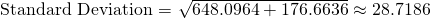Try It

On May 11, 2013 at 9:30 PM, the probability that moderate seismic activity (one moderate earthquake) would occur in the next 48 hours in Japan was about 1.08%. As in (Figure), you bet that a moderate earthquake will occur in Japan during this period. If you win the bet, you win ?100. If you lose the bet, you pay ?10. Let X = the amount of profit from a bet. Find the mean and standard deviation of X.

Some of the more common discrete probability functions are binomial, geometric, hypergeometric, and Poisson. Most elementary courses do not cover the geometric, hypergeometric, and Poisson. Your instructor will let you know if he or she wishes to cover these distributions.

A probability distribution function is a pattern. You try to fit a probability problem into a pattern or distribution in order to perform the necessary calculations. These distributions are tools to make solving probability problems easier. Each distribution has its own special characteristics. Learning the characteristics enables you to distinguish among the different distributions.

### References

Class Catalogue at the Florida State University. Available online at https://apps.oti.fsu.edu/RegistrarCourseLookup/SearchFormLegacy (accessed May 15, 2013).

“World Earthquakes: Live Earthquake News and Highlights,” World Earthquakes, 2012. http://www.world-earthquakes.com/index.php?option=ethq_prediction (accessed May 15, 2013).

### Chapter Review

The expected value, or mean, of a discrete random variable predicts the long-term results of a statistical experiment that has been repeated many times. The standard deviation of a probability distribution is used to measure the variability of possible outcomes.

### Formula Review

Mean or Expected Value: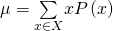Standard Deviation: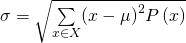Complete the expected value table.

x P(x) x*P(x)
0 0.2
1 0.2
2 0.4
3 0.2

<!– <solution id=”fs-idm67031296″>

–>

Find the expected value from the expected value table.

x P(x) x*P(x)
2 0.1 2(0.1) = 0.2
4 0.3 4(0.3) = 1.2
6 0.4 6(0.4) = 2.4
8 0.2 8(0.2) = 1.6

0.2 + 1.2 + 2.4 + 1.6 = 5.4

Find the standard deviation.

x P(x) x*P(x) (xμ)2P(x)
2 0.1 2(0.1) = 0.2 (2–5.4)2(0.1) = 1.156
4 0.3 4(0.3) = 1.2 (4–5.4)2(0.3) = 0.588
6 0.4 6(0.4) = 2.4 (6–5.4)2(0.4) = 0.144
8 0.2 8(0.2) = 1.6 (8–5.4)2(0.2) = 1.352

<!– <solution id=”fs-idm40043568″> σ= 1.156+0.588+0.144+1.352 = 3.24 =1.8 –>

Identify the mistake in the probability distribution table.

x P(x) x*P(x)
1 0.15 0.15
2 0.25 0.50
3 0.30 0.90
4 0.20 0.80
5 0.15 0.75

The values of P(x) do not sum to one.

Identify the mistake in the probability distribution table.

x P(x) x*P(x)
1 0.15 0.15
2 0.25 0.40
3 0.25 0.65
4 0.20 0.85
5 0.15 1

<!– <solution id=”fs-idp3065552″> The values of xP(x) are not correct. –>

Use the following information to answer the next five exercises: A physics professor wants to know what percent of physics majors will spend the next several years doing post-graduate research. He has the following probability distribution.

x P(x) x*P(x)
1 0.35
2 0.20
3 0.15
4
5 0.10
6 0.05

Define the random variable X.

Let X = the number of years a physics major will spend doing post-graduate research.

Define P(x), or the probability of x.

<!– <solution id=”fs-idm55979056″> Let P(x) = the probability that a physics major will do post-graduate research for x years. –>

Find the probability that a physics major will do post-graduate research for four years. P(x = 4) = _______

1 – 0.35 – 0.20 – 0.15 – 0.10 – 0.05 = 0.15

FInd the probability that a physics major will do post-graduate research for at most three years. P(x ≤ 3) = _______

<!– <solution id=”fs-idp5192912″> 0.35 + 0.20 + 0.15 = 0.70 –>

On average, how many years would you expect a physics major to spend doing post-graduate research?

1(0.35) + 2(0.20) + 3(0.15) + 4(0.15) + 5(0.10) + 6(0.05) = 0.35 + 0.40 + 0.45 + 0.60 + 0.50 + 0.30 = 2.6 years

Use the following information to answer the next seven exercises: A ballet instructor is interested in knowing what percent of each year’s class will continue on to the next, so that she can plan what classes to offer. Over the years, she has established the following probability distribution.

• Let X = the number of years a student will study ballet with the teacher.
• Let P(x) = the probability that a student will study ballet x years.

Complete (Figure) using the data provided.

x P(x) x*P(x)
1 0.10
2 0.05
3 0.10
4
5 0.30
6 0.20
7 0.10

In words, define the random variable X.

X is the number of years a student studies ballet with the teacher.

P(x = 4) = _______

<!– <solution id=”fs-idp93530752″> 1 – 0.10 – 0.05 – 0.10 – 0.30 – 0.20 – 0.10 = 0.15 –>

P(x < 4) = _______

0.10 + 0.05 + 0.10 = 0.25

On average, how many years would you expect a child to study ballet with this teacher?

<!– <solution id=”fs-idp209095920″> 1(0.10) + 2(0.05) + 3(0.10) + 4(0.15) + 5(0.30) + 6(0.20) + 7(0.10) = 4.5 years –>

What does the column “P(x)” sum to and why?

The sum of the probabilities sum to one because it is a probability distribution.

What does the column “x*P(x)” sum to and why?

<!– <solution id=”fs-idp175794960″> The sum of xP(x) = 4.5; it is the mean of the distribution. –>

You are playing a game by drawing a card from a standard deck and replacing it. If the card is a face card, you win ?30. If it is not a face card, you pay ?2. There are 12 face cards in a deck of 52 cards. What is the expected value of playing the game?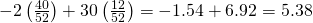You are playing a game by drawing a card from a standard deck and replacing it. If the card is a face card, you win ?30. If it is not a face card, you pay ?2. There are 12 face cards in a deck of 52 cards. Should you play the game?

<!– <solution id=”fs-idm123320016″> Yes, because there is a positive expected value, and the more you play, the more likely you are to get closer to the expected value. –>

### HOMEWORK

A theater group holds a fund-raiser. It sells 100 raffle tickets for ?5 apiece. Suppose you purchase four tickets. The prize is two passes to a Broadway show, worth a total of ?150.

1. What are you interested in here?
2. In words, define the random variable X.
3. List the values that X may take on.
4. Construct a PDF.
5. If this fund-raiser is repeated often and you always purchase four tickets, what would be your expected average winnings per raffle?

<!– <solution id=”fs-idp146807296″> I am interested in the average profit or loss. Let X = the return from the raffle Win(💲150) or Lose (💲0)

150( 1 100 )+0( 99 100 )−20=−💲18.50 –>

A game involves selecting a card from a regular 52-card deck and tossing a coin. The coin is a fair coin and is equally likely to land on heads or tails.

• If the card is a face card, and the coin lands on Heads, you win ?6
• If the card is a face card, and the coin lands on Tails, you win ?2
• If the card is not a face card, you lose ?2, no matter what the coin shows.
1. Find the expected value for this game (expected net gain or loss).
3. Should you play this game to win money?

The variable of interest is X, or the gain or loss, in dollars.

The face cards jack, queen, and king. There are (3)(4) = 12 face cards and 52 – 12 = 40 cards that are not face cards.

We first need to construct the probability distribution for X. We use the card and coin events to determine the probability for each outcome, but we use the monetary value of X to determine the expected value.

Card Event X net gain/loss P(X)
Face Card and Heads 6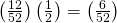Face Card and Tails 2(Not Face Card) and (H or T) –2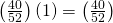•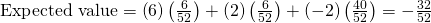• Expected value = –?0.62, rounded to the nearest cent
• If you play this game repeatedly, over a long string of games, you would expect to lose 62 cents per game, on average.
• You should not play this game to win money because the expected value indicates an expected average loss.

You buy a lottery ticket to a lottery that costs ?10 per ticket. There are only 100 tickets available to be sold in this lottery. In this lottery there are one ?500 prize, two ?100 prizes, and four ?25 prizes. Find your expected gain or loss.

<!– <solution id=”eip-id1170585488797″> Start by writing the probability distribution. X is net gain or loss = prize (if any) less 💲10 cost of ticket

Expected Value =(490)( 1 100 )+(90)( 2 100 )+(15)( 4 100 )+(−10)( 93 100 )=−💲2. There is an expected loss of 💲2 per ticket, on average. –>

Complete the PDF and answer the questions.

x P(x) xP(x)
0 0.3
1 0.2
2
3 0.4
1. Find the probability that x = 2.
2. Find the expected value.
1. 0.1
2. 1.6

Suppose that you are offered the following “deal.” You roll a die. If you roll a six, you win ?10. If you roll a four or five, you win ?5. If you roll a one, two, or three, you pay ?6.

1. What are you ultimately interested in here (the value of the roll or the money you win)?
2. In words, define the Random Variable X.
3. List the values that X may take on.
4. Construct a PDF.
5. Over the long run of playing this game, what are your expected average winnings per game?
6. Based on numerical values, should you take the deal? Explain your decision in complete sentences.

<!– <solution id=”fs-idp133159792″> the money won X = the amount of money won or lost 💲5, –💲6, 💲10

Expected Value = (10) 1 6 + (5) 2 6 – (6) 3 6 = 0.33 Yes, the expected value is 33 cents –>

A venture capitalist, willing to invest ?1,000,000, has three investments to choose from. The first investment, a software company, has a 10% chance of returning ?5,000,000 profit, a 30% chance of returning ?1,000,000 profit, and a 60% chance of losing the million dollars. The second company, a hardware company, has a 20% chance of returning ?3,000,000 profit, a 40% chance of returning ?1,000,000 profit, and a 40% chance of losing the million dollars. The third company, a biotech firm, has a 10% chance of returning ?6,000,000 profit, a 70% of no profit or loss, and a 20% chance of losing the million dollars.

1. Construct a PDF for each investment.
2. Find the expected value for each investment.
3. Which is the safest investment? Why do you think so?
4. Which is the riskiest investment? Why do you think so?
5. Which investment has the highest expected return, on average?
1. Software Company
x P(x)
5,000,000 0.10
1,000,000 0.30
–1,000,000 0.60
Hardware Company
x P(x)
3,000,000 0.20
1,000,000 0.40
–1,000,00 0.40
Biotech Firm
x P(x)
6,00,000 0.10
0 0.70
–1,000,000 0.20
2. ?200,000; ?600,000; ?400,000
3. third investment because it has the lowest probability of loss
4. first investment because it has the highest probability of loss
5. second investment

Suppose that 20,000 married adults in the United States were randomly surveyed as to the number of children they have. The results are compiled and are used as theoretical probabilities. Let X = the number of children married people have.

x P(x) xP(x)
0 0.10
1 0.20
2 0.30
3
4 0.10
5 0.05
6 (or more) 0.05
1. Find the probability that a married adult has three children.
2. In words, what does the expected value in this example represent?
3. Find the expected value.
4. Is it more likely that a married adult will have two to three children or four to six children? How do you know?

<!– <solution id=”eip-idp44220400″> 0.2 The average number of children married adults have. 2.35 two of three children –>

Suppose that the PDF for the number of years it takes to earn a Bachelor of Science (B.S.) degree is given as in (Figure).

x P(x)
3 0.05
4 0.40
5 0.30
6 0.15
7 0.10

On average, how many years do you expect it to take for an individual to earn a B.S.?

4.85 years

People visiting video rental stores often rent more than one DVD at a time. The probability distribution for DVD rentals per customer at Video To Go is given in the following table. There is a five-video limit per customer at this store, so nobody ever rents more than five DVDs.

x P(x)
0 0.03
1 0.50
2 0.24
3
4 0.07
5 0.04
1. Describe the random variable X in words.
2. Find the probability that a customer rents three DVDs.
3. Find the probability that a customer rents at least four DVDs.
4. Find the probability that a customer rents at most two DVDs.
Another shop, Entertainment Headquarters, rents DVDs and video games. The probability distribution for DVD rentals per customer at this shop is given as follows. They also have a five-DVD limit per customer.
x P(x)
0 0.35
1 0.25
2 0.20
3 0.10
4 0.05
5 0.05
5. At which store is the expected number of DVDs rented per customer higher?
6. If Video to Go estimates that they will have 300 customers next week, how many DVDs do they expect to rent next week? Answer in sentence form.
7. If Video to Go expects 300 customers next week, and Entertainment HQ projects that they will have 420 customers, for which store is the expected number of DVD rentals for next week higher? Explain.
8. Which of the two video stores experiences more variation in the number of DVD rentals per customer? How do you know that?

<!– <solution id=”fs-idp138954192″> X = the number of video rentals per costumer 0.12 0.11 0.77 Video To Go (1.82 expected value vs. 1.4 for Entertainment Headquarters) The expected number of videos rented to 300 Video To Go customers is 546. The expected number of videos rented to 420 Entertainment Headquarters customers is 588. Entertainment Headquarters will rent more videos. The standard deviation for the number of videos rented at Video To Go is 1.1609. The standard deviation for the number of videos rented at Entertainment Headquarters is 1.4293. Entertainment Headquarters has more variation. –>

<!–Review Questions??–>

A “friend” offers you the following “deal.” For a ?10 fee, you may pick an envelope from a box containing 100 seemingly identical envelopes. However, each envelope contains a coupon for a free gift.

• Ten of the coupons are for a free gift worth ?6.
• Eighty of the coupons are for a free gift worth ?8.
• Six of the coupons are for a free gift worth ?12.
• Four of the coupons are for a free gift worth ?40.

Based upon the financial gain or loss over the long run, should you play the game?

1. Yes, I expect to come out ahead in money.
2. No, I expect to come out behind in money.
3. It doesn’t matter. I expect to break even.

b

Florida State University has 14 statistics classes scheduled for its Summer 2013 term. One class has space available for 30 students, eight classes have space for 60 students, one class has space for 70 students, and four classes have space for 100 students.

1. What is the average class size assuming each class is filled to capacity?
2. Space is available for 980 students. Suppose that each class is filled to capacity and select a statistics student at random. Let the random variable X equal the size of the student’s class. Define the PDF for X.
3. Find the mean of X.
4. Find the standard deviation of X.

<!– <solution id=”fs-idm42546736″> The average class size is:  30+8(60)+70+4(100) 14 =70 P(x=30)= 1 14 P(x=60)= 8 14 P(x=70)= 1 14 P(x=100)= 4 14 Complete the following table to find the mean and standard deviation of X.

Mean of X= 30 14 + 480 14 + 70 14 + 400 14 = 980 14 =70 Standard Deviation of X= 114.2857+57.1429+0+257.1429 =20.702 –>

In a lottery, there are 250 prizes of ?5, 50 prizes of ?25, and ten prizes of ?100. Assuming that 10,000 tickets are to be issued and sold, what is a fair price to charge to break even?

Let X = the amount of money to be won on a ticket. The following table shows the PDF for X.

x P(x)
0 0.969
5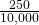= 0.025
25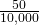= 0.005
100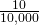= 0.001

Calculate the expected value of X.

0(0.969) + 5(0.025) + 25(0.005) + 100(0.001) = 0.35

A fair price for a ticket is ?0.35. Any price over ?0.35 will enable the lottery to raise money.

### Glossary

Expected Value
expected arithmetic average when an experiment is repeated many times; also called the mean. Notations: μ. For a discrete random variable (RV) with probability distribution function P(x),the definition can also be written in the form μ =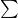xP(x).
Mean
a number that measures the central tendency; a common name for mean is ‘average.’ The term ‘mean’ is a shortened form of ‘arithmetic mean.’ By definition, the mean for a sample (detonated by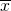) is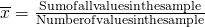and the mean for a population (denoted by μ) is μ =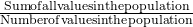.
Mean of a Probability Distribution
the long-term average of many trials of a statistical experiment
Standard Deviation of a Probability Distribution
a number that measures how far the outcomes of a statistical experiment are from the mean of the distributionThe Law of Large Numbers
As the number of trials in a probability experiment increases, the difference between the theoretical probability of an event and the relative frequency probability approaches zero.